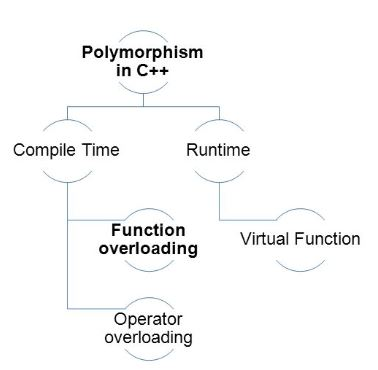×

Search anything:FREE BOOK -> Problems for the day before your Coding Interview (on Amazon)

Reading time: 20 minutes | Coding time: 2 minutes

Function overloading is a feature in C++ where two or more functions can have the same name but different parameters. In other words the signature (data type and order) can be different but the function name should be same. The return type does not really affect it.

Function overloading can be considered as an example of static time polymorphism feature in C++.

This is used in situations when a class B is inherits from class A and a particular behaviour of the class B needs to be modified. In this case, the particular function in class B needs to overload the function of class A.Example

``````#include <iostream>
using namespace std;

void print(int i)
{
cout << "int " << i << endl;
}
void print(double  f)
{
cout << "float " << f << endl;
}
void print(char const *c)
{
cout << "char* " << c << endl;
}

int main()
{
print(10);
print(10.10);
print("ten");
return 0;
}
``````

Output:

``````int 10
float 10.1
char* ten
``````

How does compiler decides which function to call?

Compiler differentiate between the functions by naming the function with it's own shortcut this process is called mangling.
Lets say for the above example the compiler will give following name to the functions :

``````    void print(int i)         => vpi
void print(double f)      => vpd
void print(char const *c) => vpc
``````

Note : The above example is hypothetical.
The user/programmer will never know these names as it is for the compiler convience.

### Functions that cannot be overloaded in C++

1. Function declarations that differ only in the return type. For example, the following program fails in compilation.
``````#include<iostream>
using namespace std;
int foo()
{
return 10;
}
char foo()
{
return 'a';
}
int main()
{
char x = foo();
getchar();
return 0;
}
``````
1. Member function declarations with the same name and the name parameter-type-list cannot be overloaded if any of them is a static member function declaration. For example, following program fails in compilation.
``````#include<iostream>
using namespace std;
class Test
{
static void fun(int i){}
void fun(int i) {}
};
int main()
{
Test t;
getchar();
return 0;
}
``````
1. Parameter declarations that differ only in a pointer * versus an array [] are equivalent. That is, the array declaration is adjusted to become a pointer declaration. Only the second and subsequent array dimensions are significant in parameter types. For example, following two function declarations are equivalent.
``````int fun(int *ptr);
int fun(int ptr[]); // redeclaration of fun(int *ptr)
``````
1. Parameter declarations that differ only in that one is a function type and the other is a pointer to the same function type are equivalent.
``````void h(int ());
void h(int (*)()); // redeclaration of h(int())
``````
1. Parameter declarations that differ only in the presence or absence of const and/or volatile are equivalent. That is, the const and volatile type-specifiers for each parameter type are ignored when determining which function is being declared, defined, or called. For example, following program fails in compilation with error “redefinition of `int f(int)’ “

Example:

``````#include<iostream>
using namespace std;
int f ( int x)
{
return x+10;
}

int f ( const int x)
{
return x+10;
}
int main()
{
getchar();
return 0;
}
``````
1. Two parameter declarations that differ only in their default arguments are equivalent. For example, following program fails in compilation with error “redefinition of `int f(int, int)’ “
``````#include<iostream>
using namespace std;
int f ( int x, int y)
{
return x+10;
}
int f ( int x, int y = 10)
{
return x+y;
}
int main()
{
getchar();
return 0;
}
``````

### Question

#### Which of the following overloaded functions are NOT allowed in C++?

1) Function declarations that differ only in the return type
int fun(int x, int y);
void fun(int x, int y);
2) Functions that differ only by static keyword in return type
int fun(int x, int y);
static int fun(int x, int y);
3) Parameter declarations that differ only in a pointer * versus an array []
int fun(int *ptr, int n);
int fun(int ptr[], int n);
4) Two parameter declarations that differ only in their default arguments
int fun( int x, int y);
int fun( int x, int y = 10);
All of the above
All except 2
All except 1
All except 2 and 4